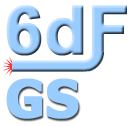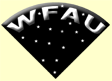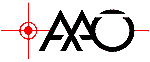* DR3 now active *
 Menu | SQL | XmatchIFA     ROE

### FITS file - 4th extension

The 4th extension holds a 2MASS H thumbnail image, the size varies with the object size.

```XTENSION= 'IMAGE   '           / IMAGE extension
BITPIX  =                  -32
NAXIS   =                    2
NAXIS1  =                   31
NAXIS2  =                   31
PCOUNT  =                    0 / required keyword; must = 0
GCOUNT  =                    1 / required keyword; must = 1
EXTNAME = '2MASS H image'      /
ORIGIN  = '2MASS   '           / 2MASS Survey Camera
TELESCOP= '2MASS   '           / North=Mt.Hopkins,South=CTIO
GID     = '004125  '           / 2MASS ID #
NNAME   = 'n/a     '           / NED Cat Name
SCANNO  = '104     '           / Scan Number
COADDNO = '186     '           / Coadd Number
ORDATE  = '990920s '           / Observation Ref Date (yymmdd)
UTDATE  = '990920  '           / UT Date of Frame (IC) (yymmdd)
UT      = '07:06:36.54'        / Time of Frame (IC) (sxgsml)
DATE-OBS= '1999-09-20T07:06:36.54' / Observation Ref Date
EQUINOX =                2000. / Equinox
CTYPE1  = 'RA---SIN'           / Orthographic Projection
CTYPE2  = 'DEC--SIN'           /
RA      =        0.03768740967 / RA  at Frame Center, J2000 (deg)
DEC     =         -46.08099365 / Dec at Frame Center, J2000 (deg)
CRVAL1  =        0.03768740967 / RA  at Frame Center, J2000 (deg)
CRVAL2  =         -46.08099365 / Dec at Frame Center, J2000 (deg)
CRPIX1  =                  16. / Axis 1 Reference Pixel
CRPIX2  =                  16. / Axis 2 Reference Pixel
CROTA2  =      -0.007425205782 / Image Twist  E of N, J2000 (deg)
CDELT1  =     -0.0002777777845 / Axis 1 Pixel Size (deg)
CDELT2  =      0.0002777777845 / Axis 2 Pixel Size (deg)
JSKYVAL =          81.16457367 / GALWORKS J Sky Measurement
JSKYSIG =         0.5749330521 / GALWORKS J Noise Measurement
HSKYVAL =          274.0104065 / GALWORKS H Sky Measurement
HSKYSIG =         0.7801685333 / GALWORKS H Noise Measurement
KSKYVAL =          542.3426514 / GALWORKS K Sky Measurement
KSKYSIG =          1.058423996 / GALWORKS K Noise Measurement
CALID   = ' V3-CALIBRATED'     / Calibration ID
JSEESH  =         0.9700000286 / Seeing J shape parameter
HSEESH  =         0.9580000043 / Seeing H shape parameter
KSEESH  =         0.9990000129 / Seeing K shape parameter
JMAGZP  =              20.8910   / Calibrated J zero point from CALMAN
HMAGZP  =              20.4510   / Calibrated H zero point from CALMAN
KMAGZP  =              19.9340   / Calibrated K zero point from CALMAN
AMASS_FC=          1.170329571 / Airmass (aprox) at this position
COMMENT > Planes 1, 2 and 3 represent the background
COMMENT > subtracted images of the J, H and Ks bands;
COMMENT > Planes 4,5,6 represent the background **and**
COMMENT > star subtracted images of the J, H and Ks bands
HISTORY > GALWORKS Version 3
HISTORY > image created by galworks,  Tom Jarrett, IPAC/Caltech/JPL
END
```

Home | Intro | Schema | Access | FITS files

WFAU, Institute for Astronomy,
Royal Observatory, Blackford Hill
Edinburgh, EH9 3HJ, UK
Tel +44 131 668 8356 (office)
or +44 131 668 8100 (switchboard)

6dfdata@aao.gov.au
26/8/2008